The Infinity Workshop - SCOPES Digital Fabrication

### Lesson Details

Subjects
Age Ranges
Topic Tags
Standards
Author

You need to login or register to bookmark/favorite this content.

### AuthorDesmond Hearne Morrey
Informal educator
Desmond is a student at Oberlin College with a major in philosophy and planned minors in creative writing and mathematics. He is interested in alternative educational forms and particularly finding alternatives for test and memorization based math education. Desmond is… Read More

### Summary

This is a lesson to help students with a background in basic adding of fractions find some wonder in mathematical exploration. We start with a review of what fractions are and how we can represent them and their variants, manipulations, etc. Then we go into repeated addition and tending toward infinity. Themes in this lesson include fractions, asymptotes, and infinity. The lesson is structured as to give students a chance to struggle on difficult problems, work together, and explore math with a focus on aesthetics rather than practicality.

### What You'll Need

Teaching materials such as a whiteboard or chalkboard

Laser Cutter or Printer

## The Instructions

### Introduction and Warm Up

Have a class discussion about what fractions are and how to use them.

First we start with a discussion about fractions, what they are and how to use them. Students should already know everything we discuss in this first step, but it’s a good warm up for what comes next. Make sure students know the basics on adding fractions with different denominators and such. Ask the class as a whole to discuss different ways we can represent numbers and therefore fractions, i.e. as shapes such as the unit square, as numerals, or as points on a line. From here move the discussion toward how operations on numbers and fractions look in these different representations.

-What are fractions?

-What is the relationship between fractions and whole numbers? (What about when the number is the same as the denominator of the fraction?)

-How do we add fractions together? What about if they have different denominators?

-What are some ways we can represent fractions visually? (i.e. pie charts)

This is a class wide discussion, and it should be more relaxed. There don’t have to be right and wrong answers, but we can discuss which ideas are helpful/interesting and which are less so.

Ask students to find a fraction that can be added to itself infinitely many times, without reaching infinity.

As a class or in groups of 3-4, ask students to try and find a fraction that, when added to itself infinitely many times, adds to a finite number. This is an impossible task, but let students struggle for a little while. If you’re soliciting answers from the whole class maybe call on students and write ideas up on the whiteboard, and then when there are no more ideas discuss if any of them work with the whole class. If you’re having students break out into small groups, have them work on the question for a few minutes and then ask each group to put a response on the board and discuss if any of them work with the class.

Now that students have had a chance to try to find such a number, student who think there exists a fraction that could be added to itself infinitely many times and add to a finite number stand on one side of the classroom, and have students who think otherwise stand on the other side. Have each side state some reasons or arguments for their position. Let students figure this one out themselves if you can.

If you want to show them a demonstration that no single fraction can be added to itself infinitely and add to a finite number, show the following proof:

Say we want to add 1/x to itself infinitely many times, such that x is any number. We can represent such an operation like so: 1/x + 1/x + 1/x + 1/x + …

Group the numbers so that they are in groups of x fractions, each group adding to 1, since 1/x times x = 1.

Therefore, this is equivalent to adding 1 to itself infinitely many times, which tends towards infinity.

In other words, summing 1/x an infinite number of times, is the same as summing 1 an infinite number of times, and then multiplying that sum by 1/x, which is still clearly infinite.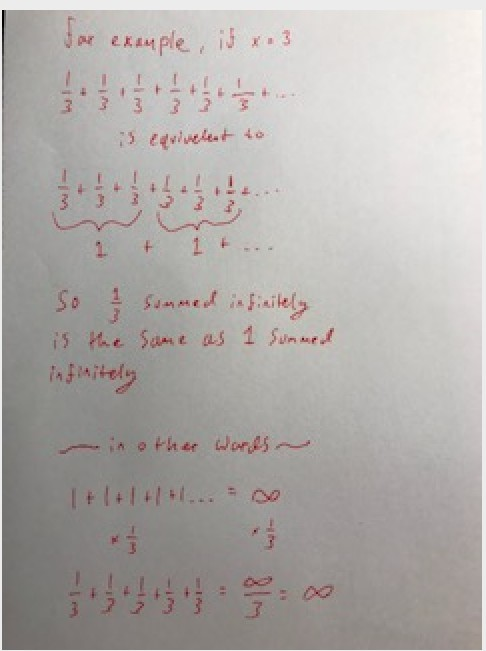Ask students to find a series of different fractions that sum to a finite number.

Now that students are (hopefully) agreed on the fact that there is no fraction that adds to a finite number after being added to itself an infinite number of times, ask students if they can find an infinite series of different fractions that add to themselves to form a finite number. Mention that, since the students obviously can’t list an infinite number of numbers to you, they’re going to need to find a pattern. An example (that does reach infinity) might be the harmonic series: 1 + 1/2 + 1/3 + 1/4 + 1/5 +… such that the denominator of the next term is always the next number on the number line. Hints could include:

-A reminder of your discussion of visual representations for fractions.

-The fact that the series of fractions is going to have to be getting smaller and smaller, since getting bigger and bigger clearly won’t work and you already tried staying the same.

-A demonstration of why the harmonic series tends toward infinity.

Have students work in groups again, (or if you had the previous discussion as a whole class, work as a whole class again). After 15 minutes (or longer if you want), have students put up their ideas on the board, and discuss each of them as a class. Discuss ways to prove that one of them works or doesn’t work. If no students have found the series 1/2^n, or 1/2+1/4+1/8+1/16+1/32+… demonstrate how it tends toward one by drawing a square, cutting it in half, then cutting one of the halves in half, and repeat so that you have something like this: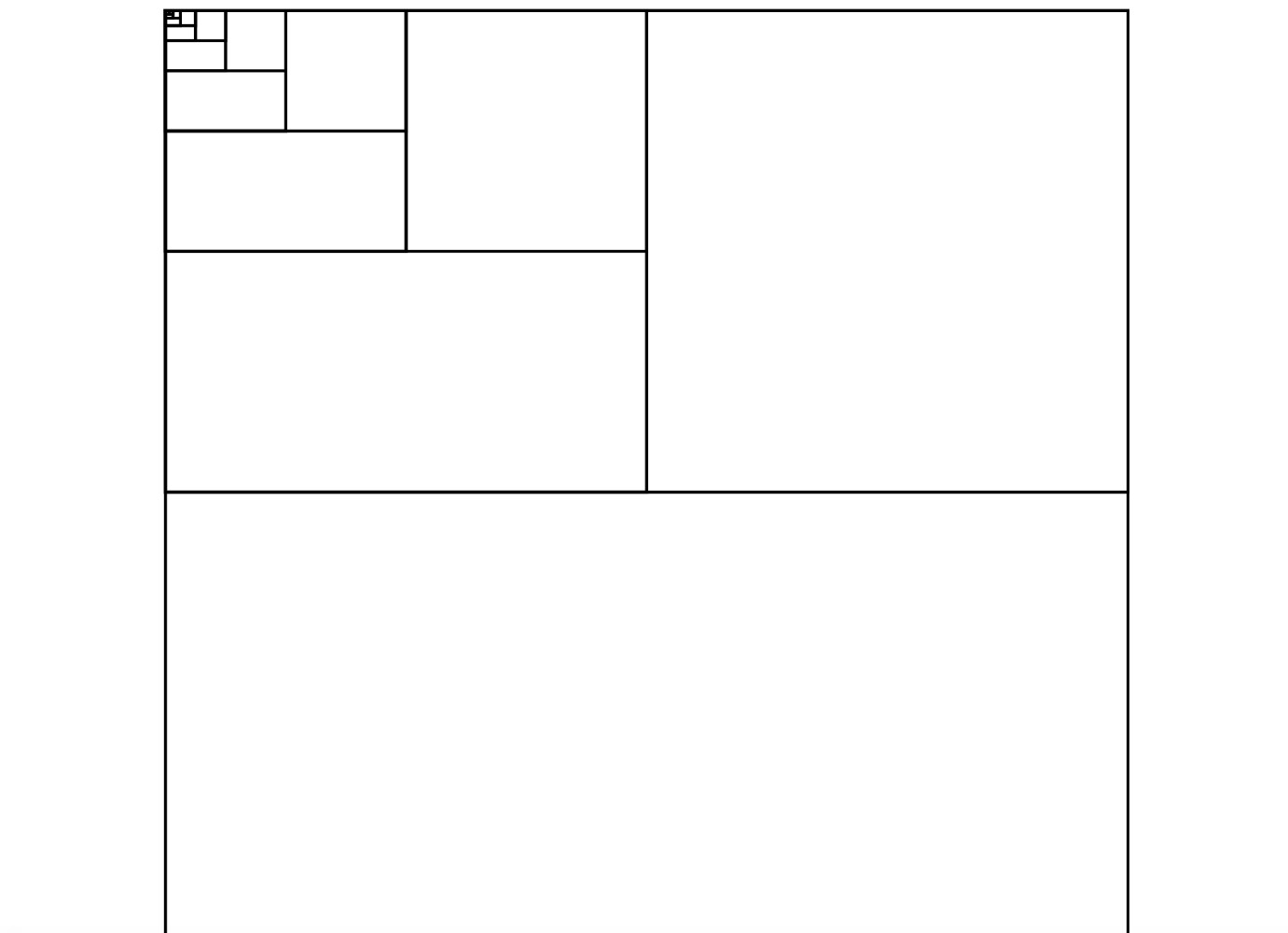If student’s weren’t able to find many series before, give them another chance. Each group )or each student, depending on how you’re structuring the lesson), should come up with a series and also a way to visually represent it within a shape. Here’s another example of the 1/2^n series, but on a circle: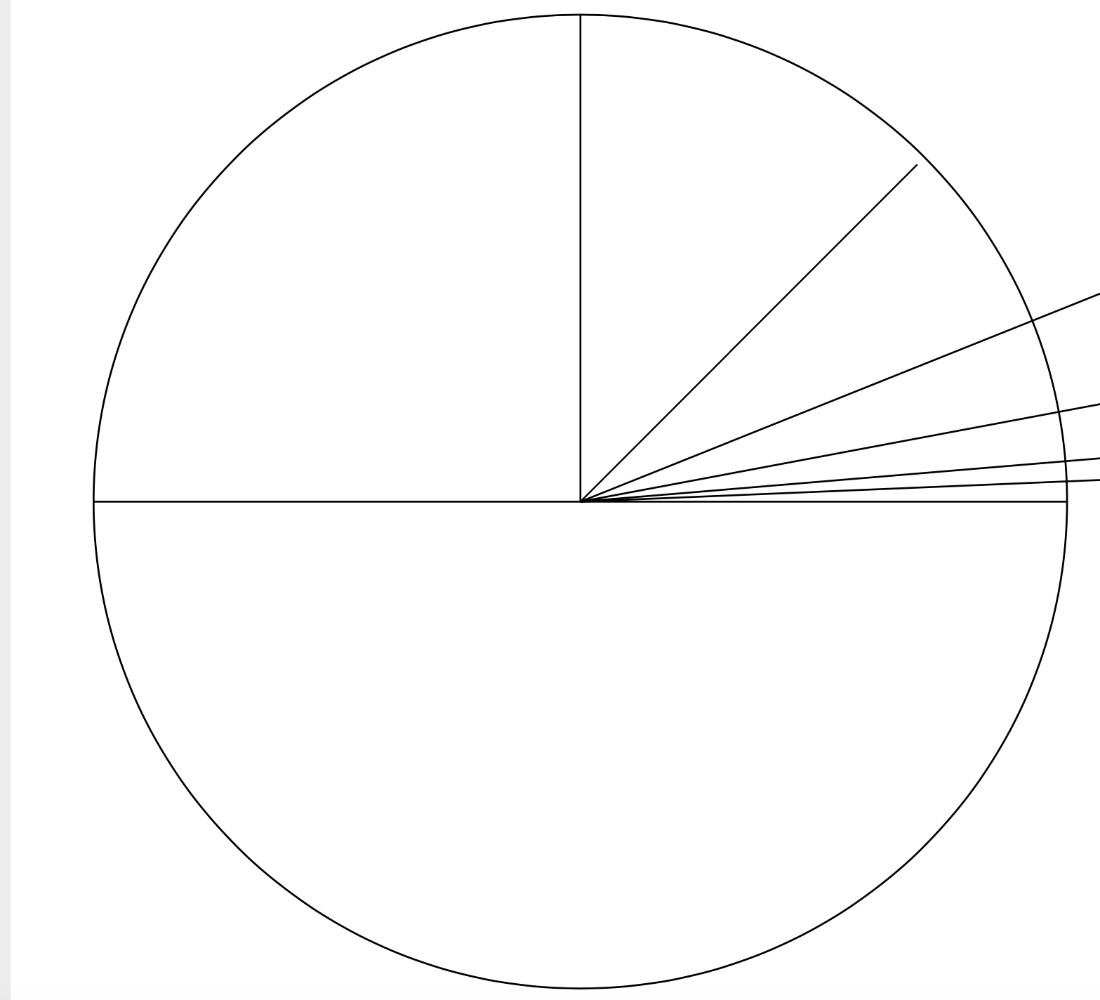### Fabrication of the Infinite

Have students put together a 2d representation of their series on the program Inkscape, and then laser cut the designs.

Students should now have a visual representation of their series drawn out with pen and paper. Now we’ll put them on Inkscape, and make cool sculptures with them! If student’s don’t know how to use Inkscape, give them a quick overview on the line tools, as well as the tools to create and edit basic shapes. If you’re looking for a refresher yourself, here’s the first video of a series of tutorials on youtube: https://www.youtube.com/watch?v=8f011wdiW7g you shouldn’t need more than the first 3 of these for this project.

Once each student has a design on Inkscape, either laser cut the designs such that there are cuts and folds where students want them, or print them on paper with a normal printer, and have students make cuts and folds themselves. This works better if you have a laser cutter, but paper, tape and scissors works okay as well. Here’s an example of one I made with the 1/2^n series: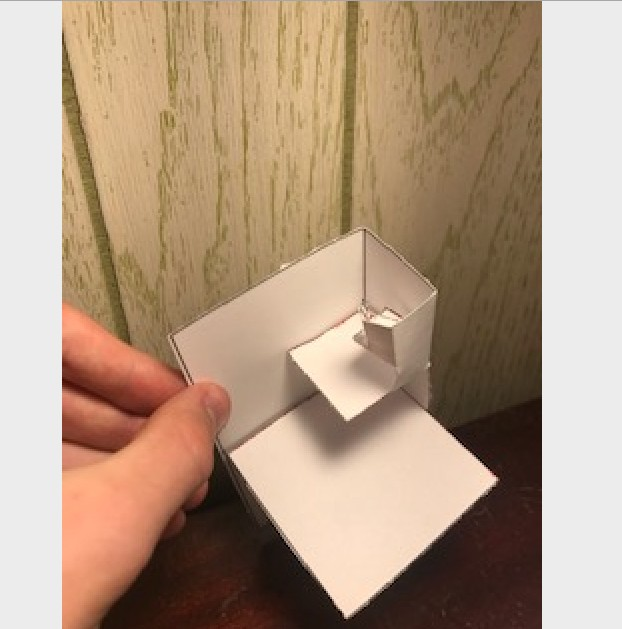Here’s where I made cuts, colored over in red and folds in blue: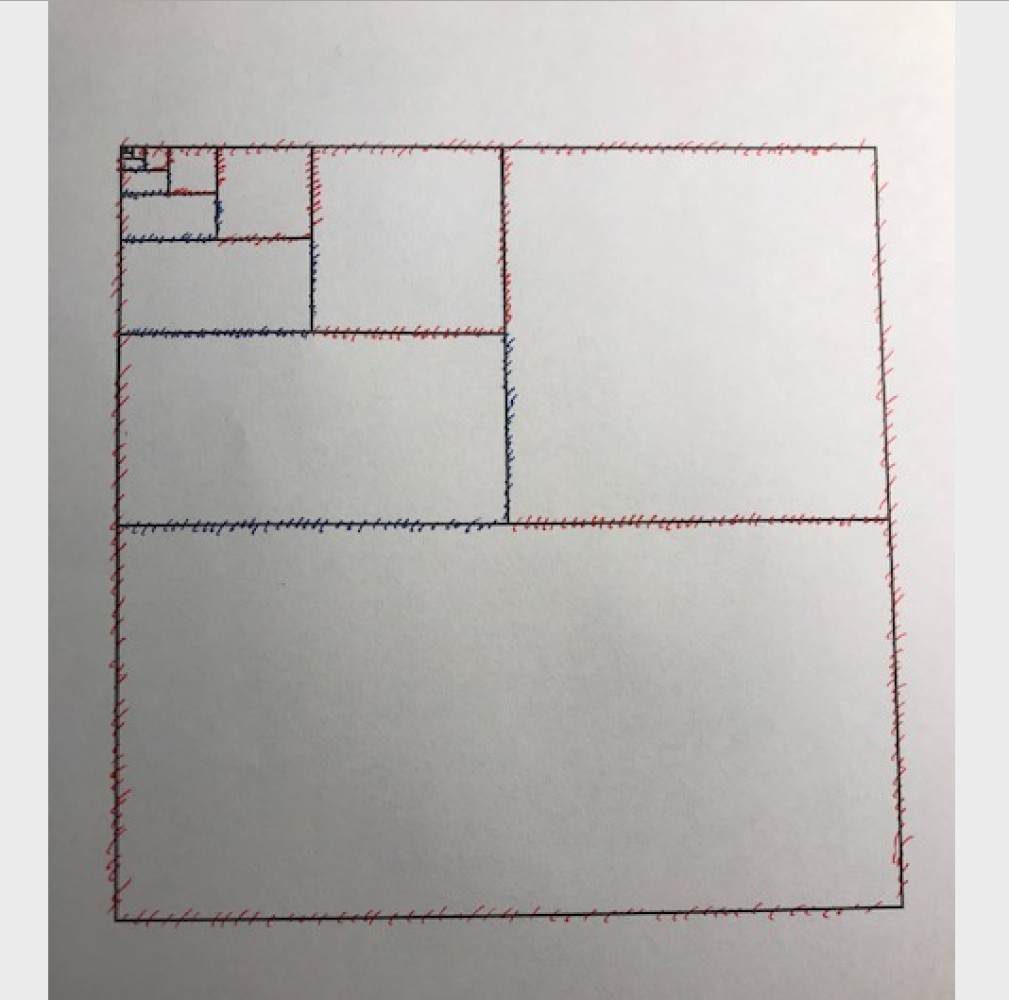## Standards

• (Fab-Safety.1): I can safely conduct myself in a Fab Lab and observe operations under instructor guidance.
• (Fab-Modeling.1): I can arrange and manipulate simple geometric elements, 2D shapes, and 3D solids using a variety of technologies.
• (Fab-Fabrication.1): I can follow instructor guided steps that link a software to a machine to produce a simple physical artifact.